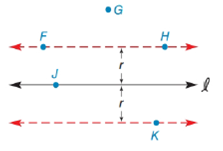Chapter 7.1, Problem 2EElementary Geometry For College St...

7th Edition
Alexander + 2 others
ISBN: 9781337614085

Solutions

Chapter
SectionElementary Geometry For College St...

7th Edition
Alexander + 2 others
ISBN: 9781337614085
Textbook Problem

Note: Exercises preceded by an asterisk are of a more challenging nature.In the figure, which of the points E ,   F ,   G ,   H , and K belong to “the locus of points in the plane that are at distance r from line l ”?To determine

To find:

The locus points that are in the plane at a distance of r from the line l from the given figure.

Explanation

Definition:

A locus is set of all points and only those points that satisfy a given condition or set of conditions.

In must be noted that, the phrase “all points and only those points” has dual meaning as follows:

1. All points of the locus satisfy the given condition.

2. All points satisfying the given locus conditions are included in the locus.

Calculation:

From the given figure,

We find that dashed line is the locus of points in the plane that are at distance r from line l

Still sussing out bartleby?

Check out a sample textbook solution.

See a sample solution

The Solution to Your Study Problems

Bartleby provides explanations to thousands of textbook problems written by our experts, many with advanced degrees!

Get Started

Find more solutions based on key concepts